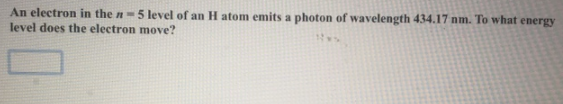Chemistry Practice Problems Bohr and Balmer Equations Practice Problems Solution: An electron in the n = 5 level of an H atom emits ...

🤓 Based on our data, we think this question is relevant for Professor Amateis' class at VT.

# Solution: An electron in the n = 5 level of an H atom emits a photon of wavelength 434.17 nm. To what energy level does the electron move?

###### Problem

An electron in the n = 5 level of an H atom emits a photon of wavelength 434.17 nm. To what energy level does the electron move?View Complete Written Solution

Bohr and Balmer Equations

Bohr and Balmer Equations

#### Q. Does a photon of visible light (λ ≈ 400 to 700 nm) have sufficient energy to excite an electron in a hydrogen atom from the n = 1 to the n = 5 energy ...

Solved • Mon Oct 15 2018 14:45:57 GMT-0400 (EDT)

Bohr and Balmer Equations

#### Q. Calculate the longest and shortest wavelengths of light emitted by electrons in the hydrogen atom that begin in the n = 6 state and then fall to state...

Solved • Mon Oct 15 2018 14:40:48 GMT-0400 (EDT)

Bohr and Balmer Equations

#### Q. Calculate the wavelength of light emitted when the following transition occur in the hydrogen atom. n = 4 → n = 3What type of electromagnetic radiatio...

Solved • Mon Oct 15 2018 14:29:57 GMT-0400 (EDT)

Bohr and Balmer Equations

#### Q. Calculate the wavelength of light produced if an electron moves from n = 5 state to n = 3 state of an electron in a hydrogen atom.Express your answer ...

Solved • Fri Oct 05 2018 13:00:11 GMT-0400 (EDT)